# 快速排序¶

「快速排序 Quick Sort」是一种基于 “分治思想” 的排序算法，速度很快、应用很广。

1. 以数组最左端元素作为基准数，初始化两个指针 i , j 指向数组两端；
2. 设置一个循环，每轮中使用 i / j 分别寻找首个比基准数大 / 小的元素，并交换此两元素；
3. 不断循环步骤 2. ，直至 i , j 相遇时跳出，最终把基准数交换至两个子数组的分界线；

「哨兵划分」执行完毕后，原数组被划分成两个部分，即 左子数组右子数组 ，且满足 左子数组任意元素 < 基准数 < 右子数组任意元素。因此，接下来我们只需要排序两个子数组即可。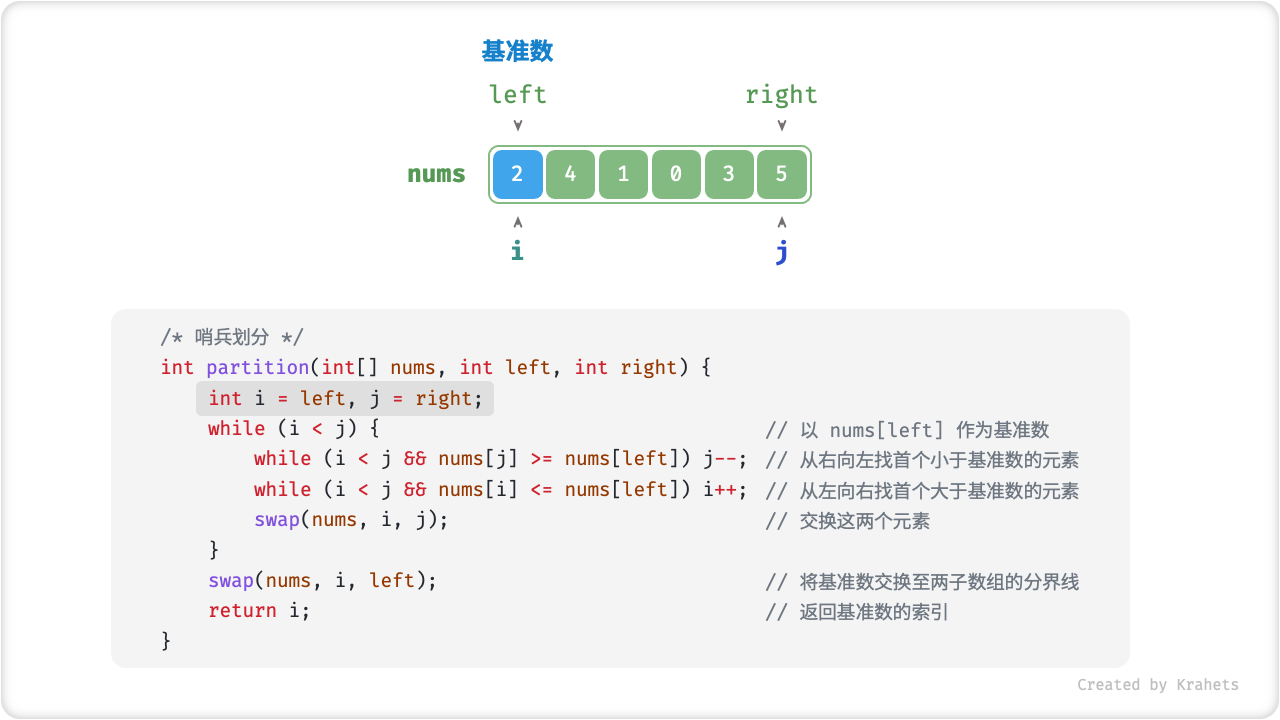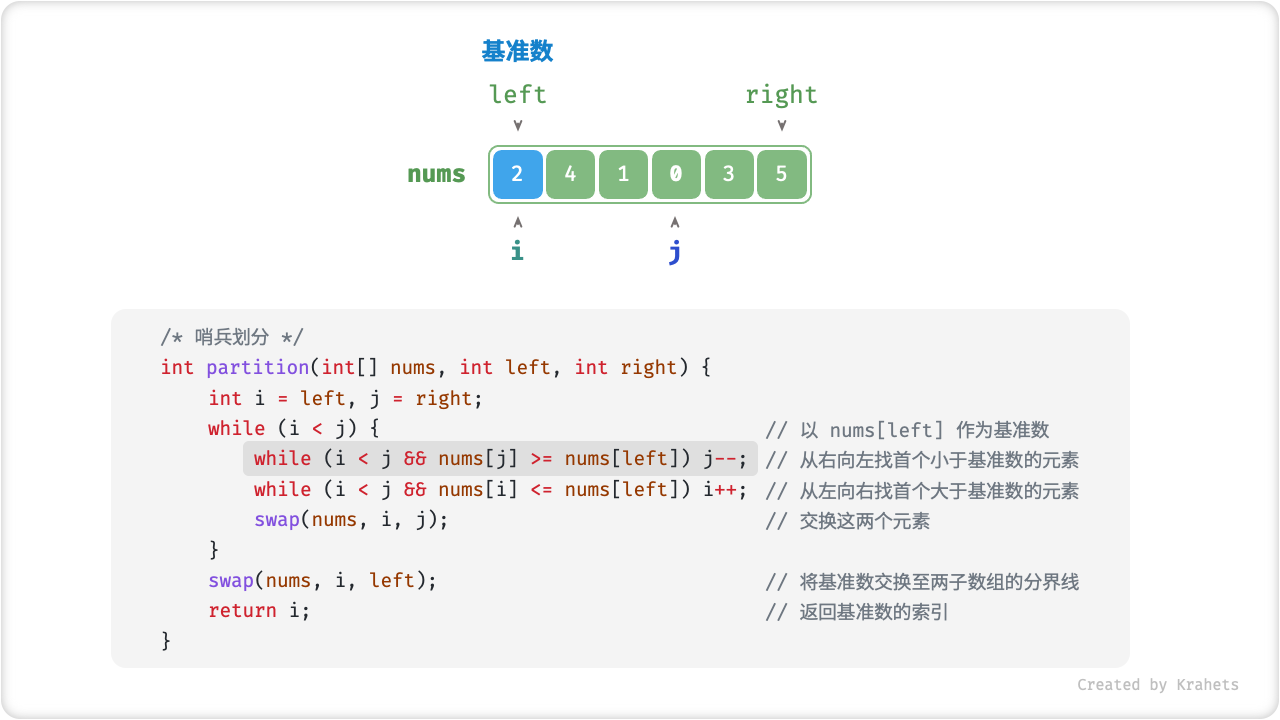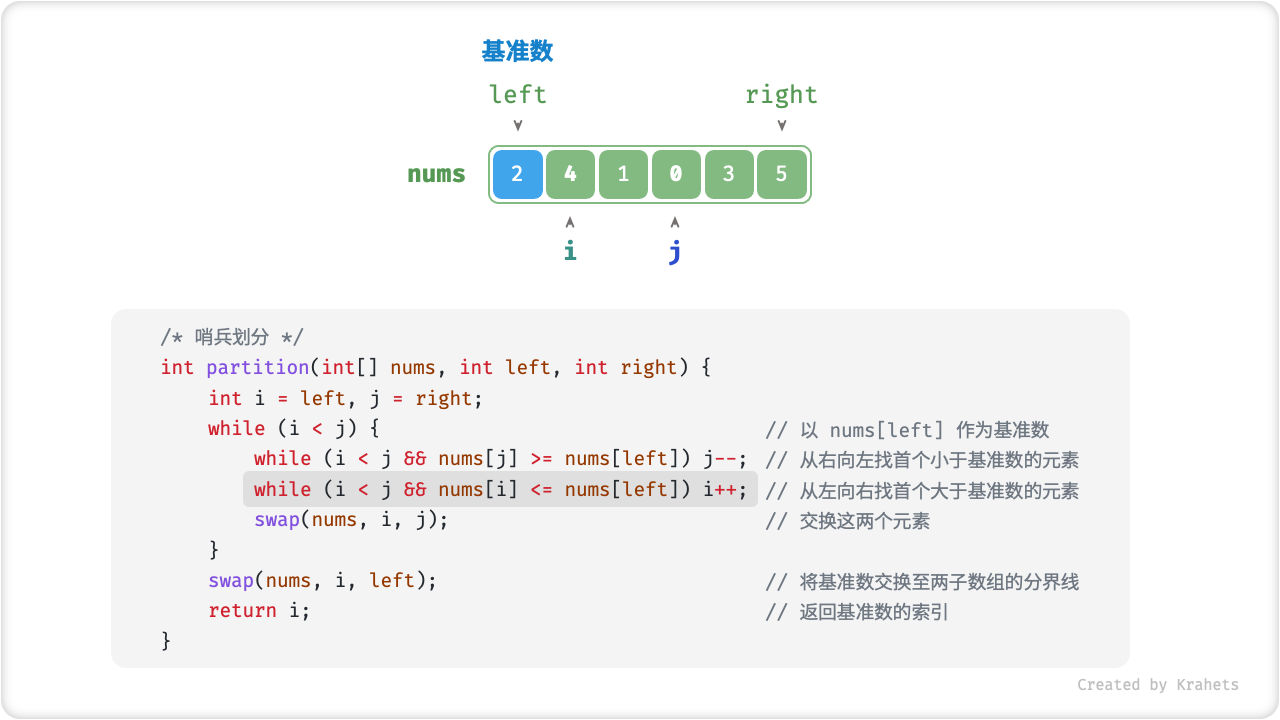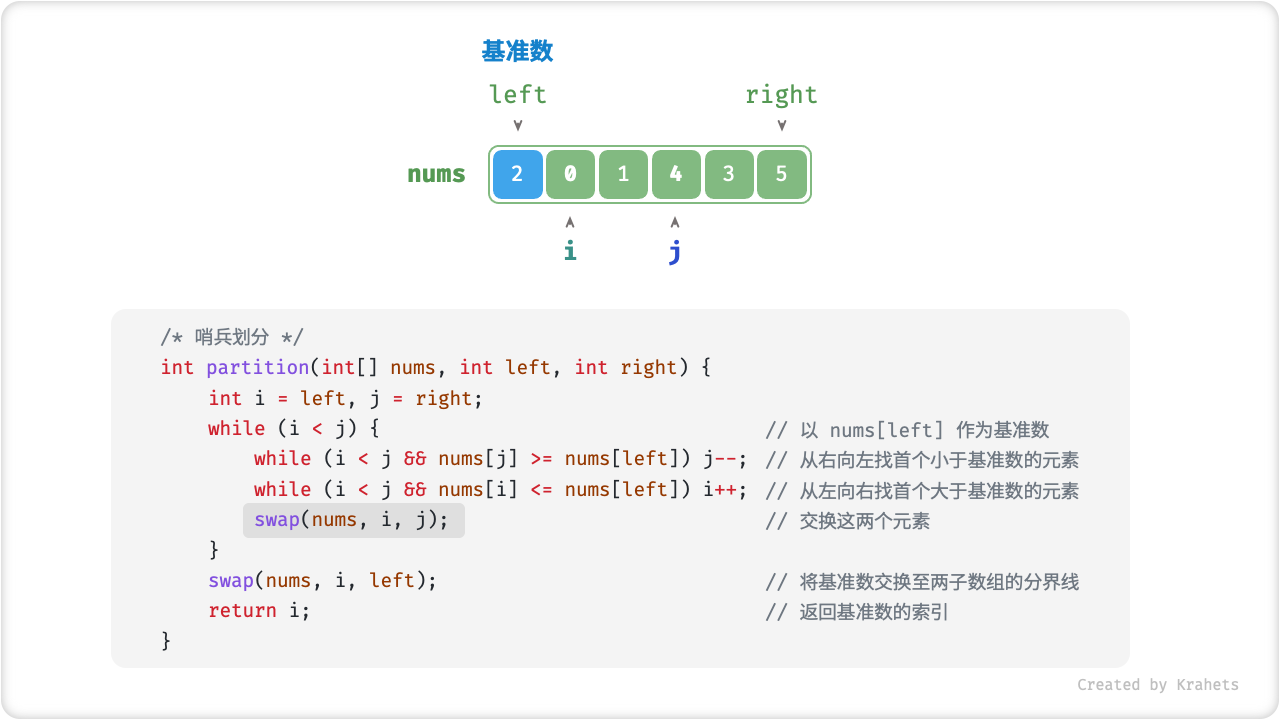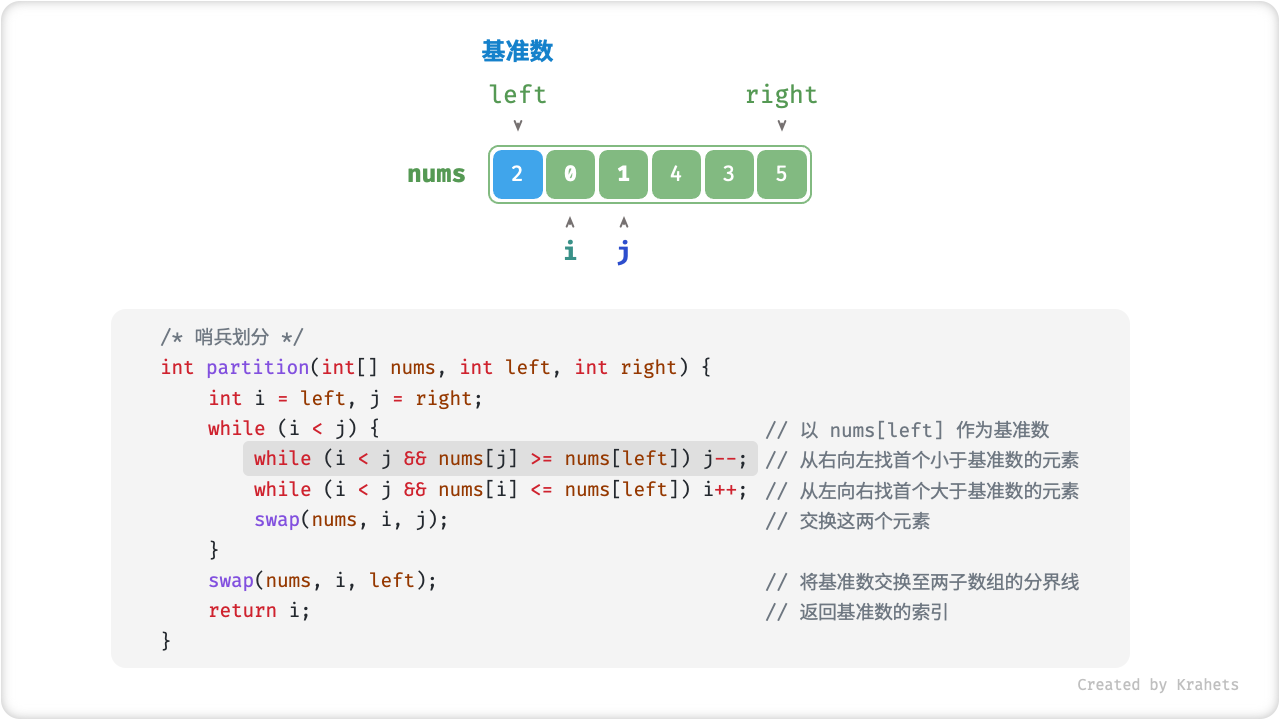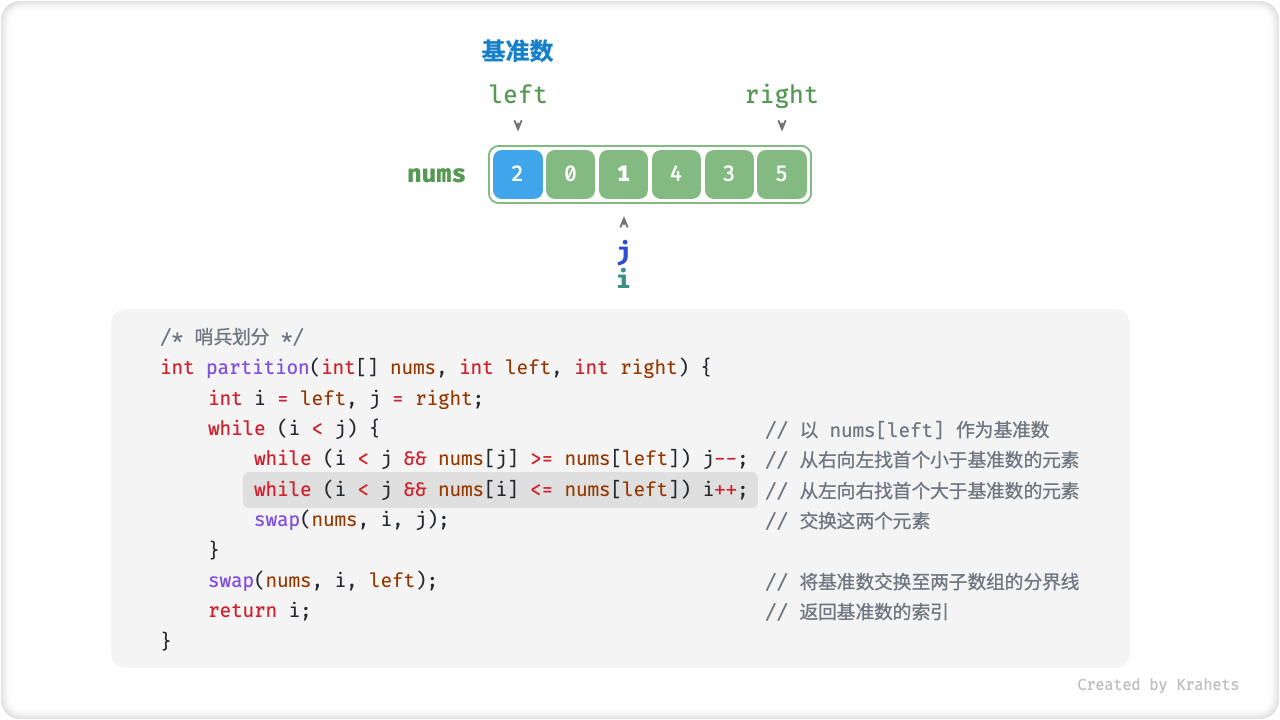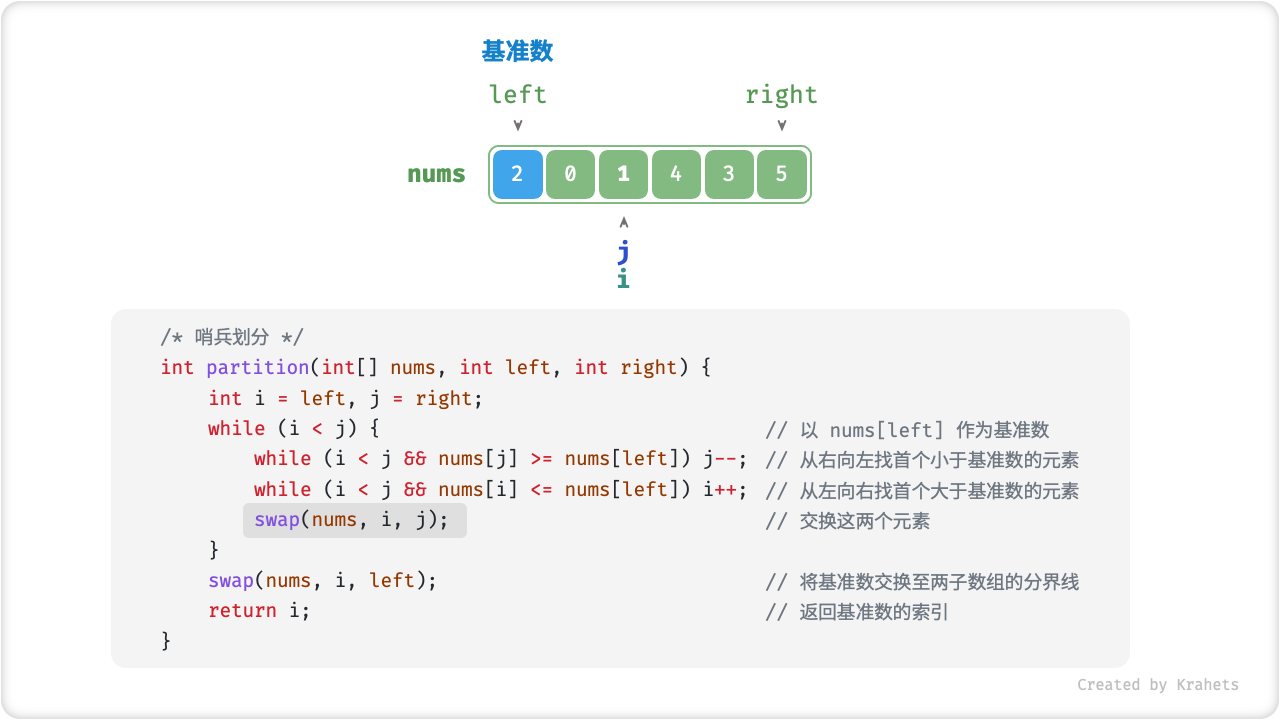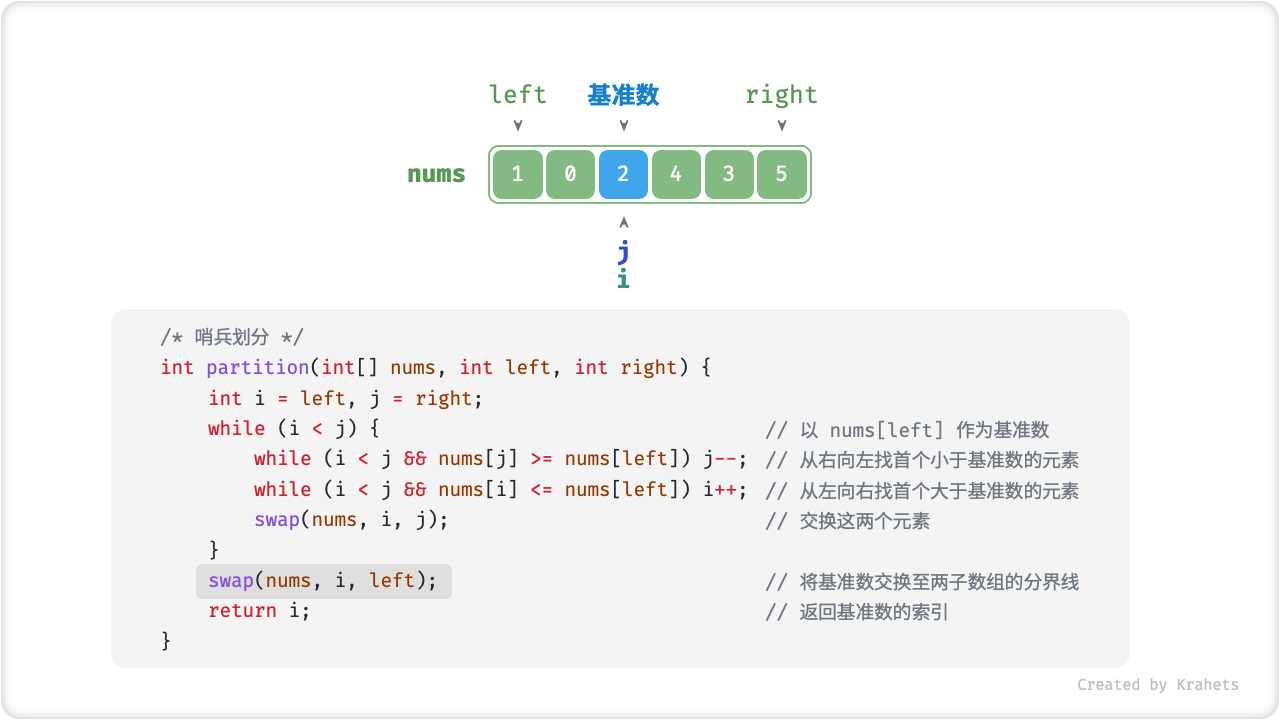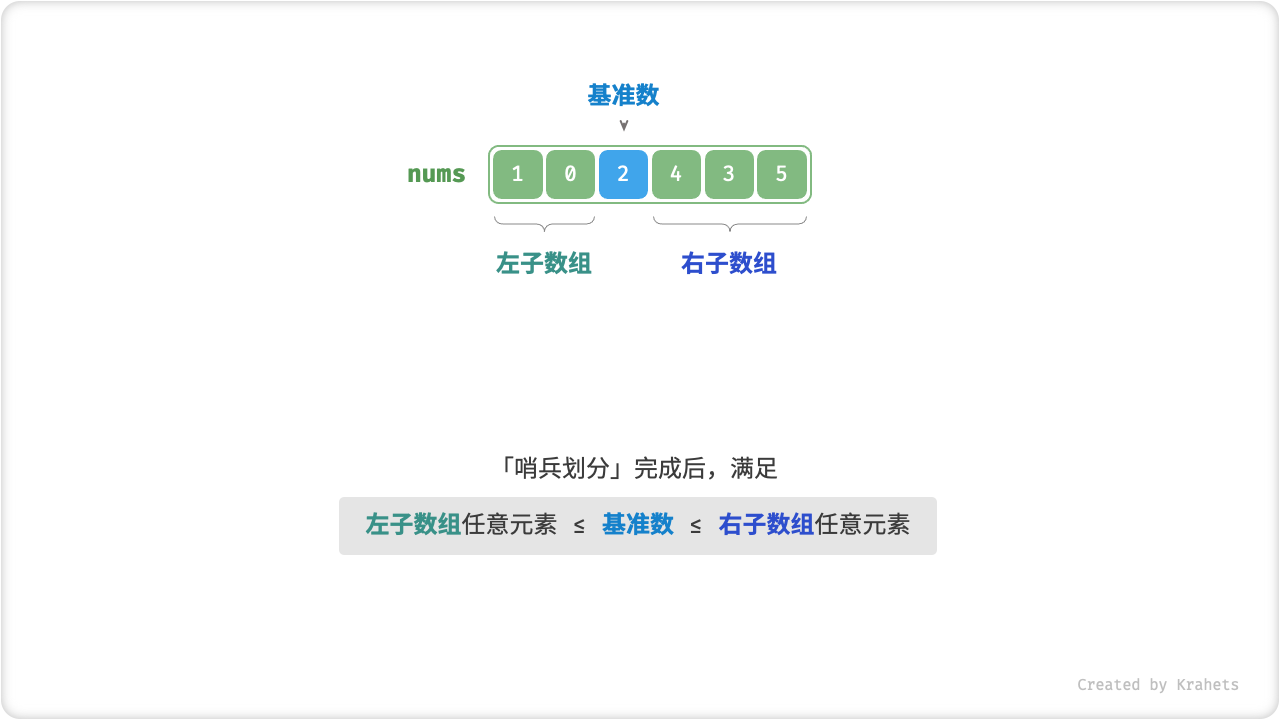Fig. 哨兵划分

quick_sort.java
/* 元素交换 */
void swap(int[] nums, int i, int j) {
int tmp = nums[i];
nums[i] = nums[j];
nums[j] = tmp;
}

/* 哨兵划分 */
int partition(int[] nums, int left, int right) {
// 以 nums[left] 作为基准数
int i = left, j = right;
while (i < j) {
while (i < j && nums[j] >= nums[left])
j--;          // 从右向左找首个小于基准数的元素
while (i < j && nums[i] <= nums[left])
i++;          // 从左向右找首个大于基准数的元素
swap(nums, i, j); // 交换这两个元素
}
swap(nums, i, left);  // 将基准数交换至两子数组的分界线
return i;             // 返回基准数的索引
}

quick_sort.cpp
/* 元素交换 */
void swap(vector<int>& nums, int i, int j) {
int tmp = nums[i];
nums[i] = nums[j];
nums[j] = tmp;
}

/* 哨兵划分 */
int partition(vector<int>& nums, int left, int right) {
// 以 nums[left] 作为基准数
int i = left, j = right;
while (i < j) {
while (i < j && nums[j] >= nums[left])
j--;          // 从右向左找首个小于基准数的元素
while (i < j && nums[i] <= nums[left])
i++;          // 从左向右找首个大于基准数的元素
swap(nums, i, j); // 交换这两个元素
}
swap(nums, i, left);  // 将基准数交换至两子数组的分界线
return i;             // 返回基准数的索引
}

quick_sort.py
""" 哨兵划分 """
def partition(self, nums, left, right):
# 以 nums[left] 作为基准数
i, j = left, right
while i < j:
while i < j and nums[j] >= nums[left]:
j -= 1  # 从右向左找首个小于基准数的元素
while i < j and nums[i] <= nums[left]:
i += 1  # 从左向右找首个大于基准数的元素
# 元素交换
nums[i], nums[j] = nums[j], nums[i]
# 将基准数交换至两子数组的分界线
nums[i], nums[left] = nums[left], nums[i]
return i  # 返回基准数的索引

quick_sort.go
/* 哨兵划分 */
func partition(nums []int, left, right int) int {
//以 nums[left] 作为基准数
i, j := left, right
for i < j {
for i < j && nums[j] >= nums[left] {
j-- //从右向左找首个小于基准数的元素
}
for i < j && nums[i] <= nums[left] {
i++ //从左向右找首个大于基准数的元素
}
//元素交换
nums[i], nums[j] = nums[j], nums[i]
}
//将基准数交换至两子数组的分界线
nums[i], nums[left] = nums[left], nums[i]
return i //返回基准数的索引
}

quick_sort.js
/* 元素交换 */
function swap(nums, i, j) {
let tmp = nums[i]
nums[i] = nums[j]
nums[j] = tmp
}

/* 哨兵划分 */
function partition(nums, left, right){
// 以 nums[left] 作为基准数
let i = left, j = right
while(i < j){
while(i < j && nums[j] >= nums[left]){
j -= 1  // 从右向左找首个小于基准数的元素
}
while(i < j && nums[i] <= nums[left]){
i += 1  // 从左向右找首个大于基准数的元素
}
// 元素交换
swap(nums, i, j) // 交换这两个元素
}
swap(nums, i, left)  // 将基准数交换至两子数组的分界线
return i  // 返回基准数的索引
}

quick_sort.ts
/* 元素交换 */
function swap(nums: number[], i: number, j: number): void {
let tmp = nums[i];
nums[i] = nums[j];
nums[j] = tmp;
}

/* 哨兵划分 */
function partition(nums: number[], left: number, right: number): number {
// 以 nums[left] 作为基准数
let i = left, j = right;
while (i < j) {
while (i < j && nums[j] >= nums[left]) {
j -= 1; // 从右向左找首个小于基准数的元素
}
while (i < j && nums[i] <= nums[left]) {
i += 1; // 从左向右找首个大于基准数的元素
}
// 元素交换
swap(nums, i, j); // 交换这两个元素
}
swap(nums, i, left); // 将基准数交换至两子数组的分界线
return i; // 返回基准数的索引
}

quick_sort.c


quick_sort.cs



## 算法流程¶

1. 首先，对数组执行一次「哨兵划分」，得到待排序的 左子数组右子数组
2. 接下来，对 左子数组右子数组 分别 递归执行「哨兵划分」……
3. 直至子数组长度为 1 时 终止递归 ，即可完成对整个数组的排序。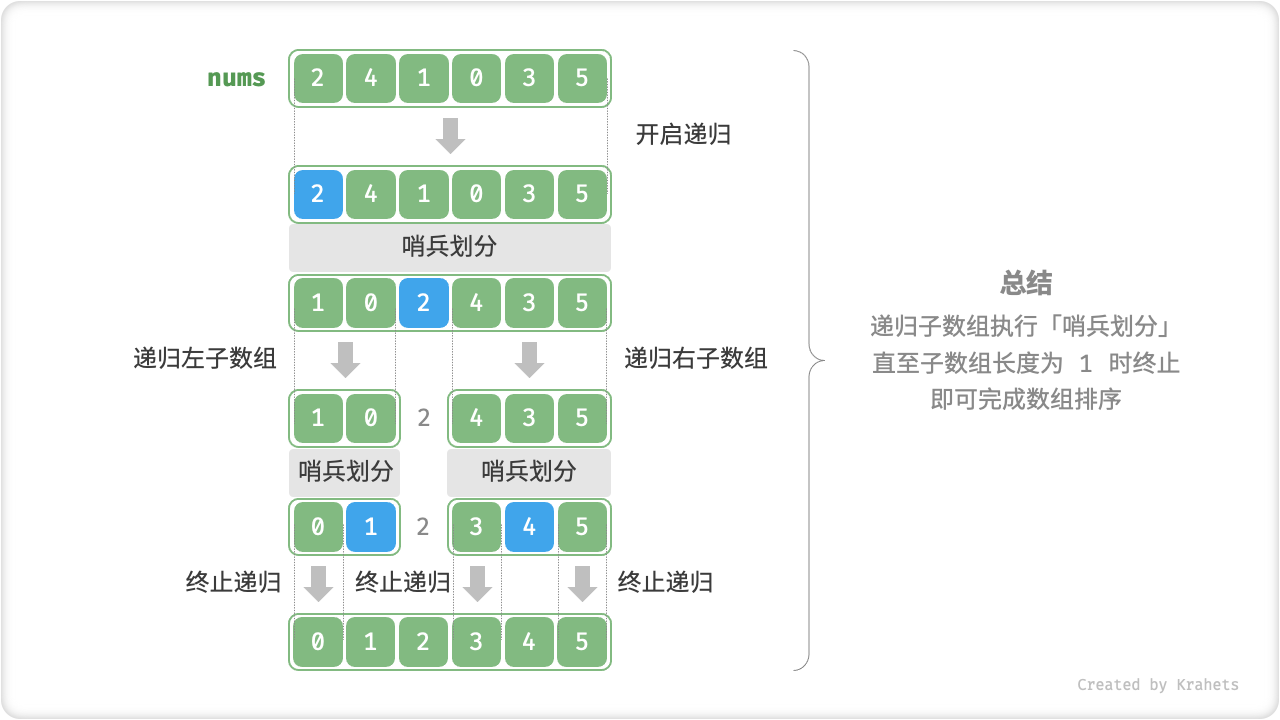Fig. 快速排序流程

quick_sort.java
/* 快速排序 */
void quickSort(int[] nums, int left, int right) {
// 子数组长度为 1 时终止递归
if (left >= right)
return;
// 哨兵划分
int pivot = partition(nums, left, right);
// 递归左子数组、右子数组
quickSort(nums, left, pivot - 1);
quickSort(nums, pivot + 1, right);
}

quick_sort.cpp
/* 快速排序 */
static void quickSort(vector<int>& nums, int left, int right) {
// 子数组长度为 1 时终止递归
if (left >= right)
return;
// 哨兵划分
int pivot = partition(nums, left, right);
// 递归左子数组、右子数组
quickSort(nums, left, pivot - 1);
quickSort(nums, pivot + 1, right);
}

quick_sort.py
""" 快速排序 """
def quick_sort(self, nums, left, right):
# 子数组长度为 1 时终止递归
if left >= right:
return
# 哨兵划分
pivot = self.partition(nums, left, right)
# 递归左子数组、右子数组
self.quick_sort(nums, left, pivot - 1)
self.quick_sort(nums, pivot + 1, right)

quick_sort.go
/* 快速排序 */
func quickSort(nums []int, left, right int) {
// 子数组长度为 1 时终止递归
if left >= right {
return
}
// 哨兵划分
pivot := partition(nums, left, right)
// 递归左子数组、右子数组
quickSort(nums, left, pivot-1)
quickSort(nums, pivot+1, right)
}

quick_sort.js
/* 快速排序 */
function quickSort(nums, left, right){
// 子数组长度为 1 时终止递归
if(left >= right) return
// 哨兵划分
const pivot = partition(nums, left, right)
// 递归左子数组、右子数组
quick_sort(nums, left, pivot - 1)
quick_sort(nums, pivot + 1, right)
}

quick_sort.ts
/* 快速排序 */
function quickSort(nums: number[], left: number, right: number): void {
// 子数组长度为 1 时终止递归
if (left >= right) {
return;
}
// 哨兵划分
const pivot = partition(nums, left, right);
// 递归左子数组、右子数组
quickSort(nums, left, pivot - 1);
quickSort(nums, pivot + 1, right);
}

quick_sort.c


quick_sort.cs



## 快排为什么快？¶

• 出现最差情况的概率很低： 虽然快速排序的最差时间复杂度为 $$O(n^2)$$ ，不如归并排序，但绝大部分情况下，快速排序可以达到 $$O(n \log n)$$ 的复杂度。
• 缓存使用效率高： 哨兵划分操作时，将整个子数组加载入缓存中，访问元素效率很高。而诸如「堆排序」需要跳跃式访问元素，因此不具有此特性。
• 复杂度的常数系数低： 在提及的三种算法中，快速排序的 比较赋值交换 三种操作的总体数量最少（类似于「插入排序」快于「冒泡排序」的原因）。

## 基准数优化¶

quick_sort.java
/* 选取三个元素的中位数 */
int medianThree(int[] nums, int left, int mid, int right) {
// 使用了异或操作来简化代码
// 异或规则为 0 ^ 0 = 1 ^ 1 = 0, 0 ^ 1 = 1 ^ 0 = 1
if ((nums[left] > nums[mid]) ^ (nums[left] > nums[right]))
return left;
else if ((nums[mid] < nums[left]) ^ (nums[mid] < nums[right]))
return mid;
else
return right;
}

/* 哨兵划分（三数取中值） */
int partition(int[] nums, int left, int right) {
// 选取三个候选元素的中位数
int med = medianThree(nums, left, (left + right) / 2, right);
// 将中位数交换至数组最左端
swap(nums, left, med);
// 以 nums[left] 作为基准数
// 下同省略...
}

quick_sort.cpp
/* 选取三个元素的中位数 */
int medianThree(vector<int>& nums, int left, int mid, int right) {
// 使用了异或操作来简化代码
// 异或规则为 0 ^ 0 = 1 ^ 1 = 0, 0 ^ 1 = 1 ^ 0 = 1
if ((nums[left] > nums[mid]) ^ (nums[left] > nums[right]))
return left;
else if ((nums[mid] < nums[left]) ^ (nums[mid] < nums[right]))
return mid;
else
return right;
}

/* 哨兵划分（三数取中值） */
int partition(vector<int>& nums, int left, int right) {
// 选取三个候选元素的中位数
int med = medianThree(nums, left, (left + right) / 2, right);
// 将中位数交换至数组最左端
swap(nums, left, med);
// 以 nums[left] 作为基准数
// 下同省略...
}

quick_sort.py
""" 选取三个元素的中位数 """
def median_three(self, nums, left, mid, right):
# 使用了异或操作来简化代码
# 异或规则为 0 ^ 0 = 1 ^ 1 = 0, 0 ^ 1 = 1 ^ 0 = 1
if (nums[left] > nums[mid]) ^ (nums[left] > nums[right]):
return left
elif (nums[mid] < nums[left]) ^ (nums[mid] > nums[right]):
return mid
return right

""" 哨兵划分（三数取中值） """
def partition(self, nums, left, right):
# 以 nums[left] 作为基准数
med = self.median_three(nums, left, (left + right) // 2, right)
# 将中位数交换至数组最左端
nums[left], nums[med] = nums[med], nums[left]
# 以 nums[left] 作为基准数
# 下同省略...

quick_sort.go
/* 选取三个元素的中位数 */
func medianThree(nums []int, left, mid, right int) int {
if (nums[left] > nums[mid]) != (nums[left] > nums[right]) {
return left
} else if (nums[mid] < nums[left]) != (nums[mid] > nums[right]) {
return mid
}
return right
}

/* 哨兵划分（三数取中值）*/
func partition(nums []int, left, right int) int {
// 以 nums[left] 作为基准数
med := medianThree(nums, left, (left+right)/2, right)
// 将中位数交换至数组最左端
nums[left], nums[med] = nums[med], nums[left]
// 以 nums[left] 作为基准数
// 下同省略...
}

quick_sort.js
/* 选取三个元素的中位数 */
function medianThree(nums, left, mid, right) {
// 使用了异或操作来简化代码
// 异或规则为 0 ^ 0 = 1 ^ 1 = 0, 0 ^ 1 = 1 ^ 0 = 1
if ((nums[left] > nums[mid]) ^ (nums[left] > nums[right]))
return left;
else if ((nums[mid] < nums[left]) ^ (nums[mid] < nums[right]))
return mid;
else
return right;
}

/* 哨兵划分（三数取中值） */
function partition(nums, left, right) {
// 选取三个候选元素的中位数
let med = medianThree(nums, left, Math.floor((left + right) / 2), right);
// 将中位数交换至数组最左端
swap(nums, left, med);
// 以 nums[left] 作为基准数
// 下同省略...
}

quick_sort.ts
/* 选取三个元素的中位数 */
function medianThree(nums: number[], left: number, mid: number, right: number): number {
// 使用了异或操作来简化代码
// 异或规则为 0 ^ 0 = 1 ^ 1 = 0, 0 ^ 1 = 1 ^ 0 = 1
if (Number(nums[left] > nums[mid]) ^ Number(nums[left] > nums[right])) {
return left;
} else if (Number(nums[mid] < nums[left]) ^ Number(nums[mid] < nums[right])) {
return mid;
} else {
return right;
}
}

/* 哨兵划分（三数取中值） */
function partition(nums: number[], left: number, right: number): number {
// 选取三个候选元素的中位数
let med = medianThree(nums, left, Math.floor((left + right) / 2), right);
// 将中位数交换至数组最左端
swap(nums, left, med);
// 以 nums[left] 作为基准数
// 下同省略...

quick_sort.c


quick_sort.cs



## 尾递归优化¶

quick_sort.java
/* 快速排序（尾递归优化） */
void quickSort(int[] nums, int left, int right) {
// 子数组长度为 1 时终止
while (left < right) {
// 哨兵划分操作
int pivot = partition(nums, left, right);
// 对两个子数组中较短的那个执行快排
if (pivot - left < right - pivot) {
quickSort(nums, left, pivot - 1);  // 递归排序左子数组
left = pivot + 1;  // 剩余待排序区间为 [pivot + 1, right]
} else {
quickSort(nums, pivot + 1, right); // 递归排序右子数组
right = pivot - 1; // 剩余待排序区间为 [left, pivot - 1]
}
}
}

quick_sort.cpp
/* 快速排序（尾递归优化） */
static void quickSort(vector<int>& nums, int left, int right) {
// 子数组长度为 1 时终止
while (left < right) {
// 哨兵划分操作
int pivot = partition(nums, left, right);
// 对两个子数组中较短的那个执行快排
if (pivot - left < right - pivot) {
quickSort(nums, left, pivot - 1);  // 递归排序左子数组
left = pivot + 1;  // 剩余待排序区间为 [pivot + 1, right]
} else {
quickSort(nums, pivot + 1, right); // 递归排序右子数组
right = pivot - 1; // 剩余待排序区间为 [left, pivot - 1]
}
}
}

quick_sort.py
""" 快速排序（尾递归优化） """
def quick_sort(self, nums, left, right):
# 子数组长度为 1 时终止
while left < right:
# 哨兵划分操作
pivot = self.partition(nums, left, right)
# 对两个子数组中较短的那个执行快排
if pivot - left < right - pivot:
self.quick_sort(nums, left, pivot - 1)  # 递归排序左子数组
left = pivot + 1     # 剩余待排序区间为 [pivot + 1, right]
else:
self.quick_sort(nums, pivot + 1, right)  # 递归排序右子数组
right = pivot - 1    # 剩余待排序区间为 [left, pivot - 1]

quick_sort.go
/* 快速排序（尾递归优化）*/
func quickSort(nums []int, left, right int) {
// 子数组长度为 1 时终止
for left < right {
// 哨兵划分操作
pivot := partition(nums, left, right)
// 对两个子数组中较短的那个执行快排
if pivot-left < right-pivot {
quickSort(nums, left, pivot-1)   // 递归排序左子数组
left = pivot + 1                 // 剩余待排序区间为 [pivot + 1, right]
} else {
quickSort(nums, pivot+1, right)  // 递归排序右子数组
right = pivot - 1                // 剩余待排序区间为 [left, pivot - 1]
}
}
}

quick_sort.js
/* 快速排序（尾递归优化） */
function quickSort(nums, left, right) {
// 子数组长度为 1 时终止
while (left < right) {
// 哨兵划分操作
let pivot = partition(nums, left, right);
// 对两个子数组中较短的那个执行快排
if (pivot - left < right - pivot) {
quickSort(nums, left, pivot - 1);  // 递归排序左子数组
left = pivot + 1;  // 剩余待排序区间为 [pivot + 1, right]
} else {
quickSort(nums, pivot + 1, right); // 递归排序右子数组
right = pivot - 1; // 剩余待排序区间为 [left, pivot - 1]
}
}
}

quick_sort.ts
/* 快速排序（尾递归优化） */
function quickSort(nums: number[], left: number, right: number): void {
// 子数组长度为 1 时终止
while (left < right) {
// 哨兵划分操作
let pivot = partition(nums, left, right);
// 对两个子数组中较短的那个执行快排
if (pivot - left < right - pivot) {
quickSort(nums, left, pivot - 1); // 递归排序左子数组
left = pivot + 1; // 剩余待排序区间为 [pivot + 1, right]
} else {
quickSort(nums, pivot + 1, right); // 递归排序右子数组
right = pivot - 1; // 剩余待排序区间为 [left, pivot - 1]
}
}
}

quick_sort.c


quick_sort.cs